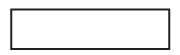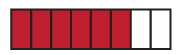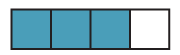### Using the area model to show equivalent fractions

We have seen that the fractions $$\dfrac{6}{8} \text{and} \dfrac{3}{4}$$ mark the same point on the number line and so are equivalent.

Equivalence can also be shown using rectangles shaded to represent each fraction.We start with a rectangle of area 1. Then we shade to show the fractions we want to compare. In each case the same area is shaded.In this rectangle 6 out of 8 equal pieces have been shaded. So we say that $$\dfrac{6}{8}$$ has been shaded.In this rectangle 3 out of 4 equal pieces have been shaded. So we say that $$\dfrac{3}{4}$$ has been shaded.